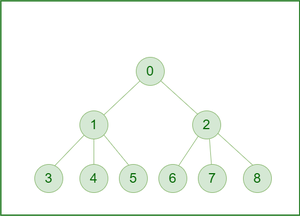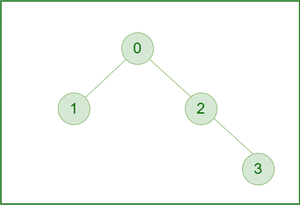Open in App
Not now

# Find most frequent value of ancestors for each Node of given Tree

• Difficulty Level : Hard
• Last Updated : 13 Mar, 2023

Given a tree with N vertices from 0 to N-1 (0th node is root) and val[] where val[i] denotes the value of the ith vertex. The task is to find the array of integers ans[] of length N, where ans[i] denotes the mode value of all its ancestors’ values including ith vertex.

Note: Mode is the value that has the highest frequency in a given set of values(if there is more than one value having the highest frequency return the largest among them).

Examples:

Input: Below is the given Tree:Example 1

val[] = [5, 2, 3, 2, 5, 1, 3, 5, 1]
Output:  5 5 5 2 5 5 3 5 5
Explanation:
node 0: {5}, mode = 5
node 1: {5, 2}, mode = 5
node 2: {5, 3}, mode = 5
node 3: {5, 2, 2}, mode = 2
node 4: {5, 2, 5}, mode = 5
node 5: {5, 2, 1}, mode = 5
node 6: {5, 3, 3}, mode = 3
node 7: {5, 3, 5}, mode = 5
node 8: {5, 3, 1}, mode = 5

Input: Below is the given Tree:Example 2

val[] = [1, 3, 2, 1]
Output: 1 3 2 1

Approach: The problem can be solved based on the following idea:

Use DFS traversal from root to all nodes in top-down manner and use a map to store the frequency of all the ancestors for a node.

Follow the steps mentioned below to implement the idea

• Use a top-down DFS to traverse from the root to all the nodes and use a map freq to store the frequency of all ancestors.
• Create a set pair s to store values and frequency of values of all ancestors in the path from the root to the current node.
• Whenever entering a node, before calling DFS on its children, increment the value of that node in freq[] and accordingly update the set s.
• Whenever we exit the node, decrement the value of that node in freq[] and accordingly update the set s
• This ensures that the set will contain the frequency of each value of all ancestors (node itself) of the current node.
• Use a map ans[] to store the required answer for each node by taking the last element of the set(highest freq value) and use that to print the final solution.

Below is the implementation of the above approach:

## C++

 `// C++ code to implement the approach`   `#include ` `using` `namespace` `std;`   `// Map to store final ans for each node` `unordered_map<``int``, ``int``> ans;`   `// Map to store frequency of values` `unordered_map<``int``, ``int``> freq;`   `// Set to store freq of value and value itself` `set > s;`   `// Function to add an edge` `// between nodes u and v` `void` `addEdge(vector<``int``> adj[], ``int` `u, ``int` `v)` `{` `    ``adj[u].push_back(v);` `    ``adj[v].push_back(u);` `}`   `// Change the values of freq and set s` `void` `helper(``int` `v, ``int` `flag)` `{` `    ``if` `(s.find({ freq[v], v }) != s.end())` `        ``s.erase(s.find({ freq[v], v }));` `    ``freq[v] += flag;` `    ``s.insert({ freq[v], v });` `}`   `// Mode of Ancestor` `void` `ModeOfAncestors(vector<``int``> adj[], ``int` `node,` `                     ``vector<``int``>& vis, vector<``int``>& val)` `{` `    ``int` `v = val[node];`   `    ``// Increment the freq of value v by one` `    ``helper(v, 1);` `    ``ans[node] = (*(--s.end())).second;` `    ``vis[node] = 1;` `    ``for` `(``auto` `child : adj[node]) {` `        ``if` `(vis[child] == 0)` `            ``ModeOfAncestors(adj, child, vis, val);` `    ``}`   `    ``// Decrement the freq of value v by one` `    ``helper(v, -1);` `}`   `// Driver Code` `int` `main()` `{` `    ``// Number of nodes in a tree` `    ``int` `N = 9;`   `    ``// Initialize tree` `    ``vector<``int``> adj[N];`   `    ``// Tree Formation` `    ``addEdge(adj, 0, 1);` `    ``addEdge(adj, 0, 2);` `    ``addEdge(adj, 1, 3);` `    ``addEdge(adj, 1, 4);` `    ``addEdge(adj, 1, 5);` `    ``addEdge(adj, 2, 6);` `    ``addEdge(adj, 2, 7);` `    ``addEdge(adj, 2, 8);`   `    ``// Values of nodes of tree` `    ``vector<``int``> val = { 5, 2, 3, 2, 5, 1, 3, 5, 1 };` `    ``vector<``int``> vis(N, 0);`   `    ``// Function call` `    ``ModeOfAncestors(adj, 0, vis, val);`   `    ``for` `(``int` `i = 0; i < N; i++)` `        ``cout << ans[i] << ``" "``;` `    ``cout << endl;`   `    ``return` `0;` `}`

## Python3

 `#Python3 code for the above approach`   `# Map to store final ans for each node` `ans ``=` `{}`   `# Map to store frequency of values` `freq ``=` `{}`     `# Set to store freq of value and value itself` `s ``=` `set``()`   `# Function to add an edge between nodes u and v` `def` `add_edge(adj, u, v):` `    ``adj[u].append(v)` `    ``adj[v].append(u)`   `# Change the values of freq and set s` `def` `helper(v, flag):` `    ``if` `(freq[v], v) ``in` `s:` `        ``s.remove((freq[v], v))` `    ``freq[v] ``+``=` `flag` `    ``s.add((freq[v], v))`   `# Mode of Ancestor` `def` `mode_of_ancestors(adj, node, vis, val):` `    ``v ``=` `val[node]`   `    ``# Increment the freq of value v by one` `    ``helper(v, ``1``)` `    ``ans[node] ``=` `max``(freq, key``=``freq.get)` `    ``vis[node] ``=` `1` `    ``for` `child ``in` `adj[node]:` `        ``if` `vis[child] ``=``=` `0``:` `            ``mode_of_ancestors(adj, child, vis, val)`   `    ``# Decrement the freq of value v by one` `    ``helper(v, ``-``1``)`     `# Number of nodes in a tree` `N ``=` `9`   `# Initialize tree` `adj ``=` `[[] ``for` `_ ``in` `range``(N)]`   `# Tree Formation` `add_edge(adj, ``0``, ``1``)` `add_edge(adj, ``0``, ``2``)` `add_edge(adj, ``1``, ``3``)` `add_edge(adj, ``1``, ``4``)` `add_edge(adj, ``1``, ``5``)` `add_edge(adj, ``2``, ``6``)` `add_edge(adj, ``2``, ``7``)` `add_edge(adj, ``2``, ``8``)`   `# Values of nodes of tree` `val ``=` `[``5``, ``2``, ``3``, ``2``, ``5``, ``1``, ``3``, ``5``, ``1``]` `vis ``=` `[``0``] ``*` `N` `for` `i, v ``in` `enumerate``(val):` `    ``freq[v] ``=` `0` `# Function call` `mode_of_ancestors(adj, ``0``, vis, val)`   `for` `i ``in` `range``(N):` `    ``print``(ans[i], end``=``" "``)` `print``()`   `#This code is contributed by Potta Lokesh`

## Javascript

 `// // JavaScript code to implement the approach`   `// Map to store final ans for each node` `let ans = {};`   `// Map to store frequency of values` `let freq = {};`   `// Set to store freq of value and value itself` `let s = ``new` `Set();`   `// Function to add an edge between nodes u and v` `function` `addEdge(adj, u, v) {` `    ``adj[u].push(v);` `    ``adj[v].push(u);` `}`   `// Change the values of freq and set s` `function` `helper(v, flag) {` `    ``if` `(s.has(`\${freq[v]},\${v}`)) {` `        ``s.``delete``(`\${freq[v]},\${v}`);` `    ``}` `    ``freq[v] += flag;` `    ``s.add(`\${freq[v]},\${v}`);` `}`   `// Mode of Ancestor` `function` `ModeOfAncestors(adj, node, vis, val) {` `    ``let v = val[node];`   `    ``// Increment the freq of value v by one` `    ``helper(v, 1);` `    ``ans[node] = Object.entries(freq).reduce((a, e) => (freq[a] > freq[e] ? a : e), [v, 0]);` `    ``vis[node] = 1;` `    ``for` `(let child of adj[node]) {` `        ``if` `(vis[child] === 0) {` `            ``ModeOfAncestors(adj, child, vis, val);` `        ``}` `    ``}`   `    ``// Decrement the freq of value v by one` `    ``helper(v, -1);` `}`   `// Driver Code`   `// Number of nodes in a tree` `const N = 9;`   `// Initialize tree` `let adj = ``new` `Array(N);` `for` `(let i = 0; i < N; i++) {` `    ``adj[i] = [];` `}`   `// Tree Formation` `addEdge(adj, 0, 1);` `addEdge(adj, 0, 2);` `addEdge(adj, 1, 3);` `addEdge(adj, 1, 4);` `addEdge(adj, 1, 5);` `addEdge(adj, 2, 6);` `addEdge(adj, 2, 7);` `addEdge(adj, 2, 8);`   `// Values of nodes of tree` `let val = [5, 2, 3, 2, 5, 1, 3, 5, 1];` `let vis = ``new` `Array(N).fill(0);` `for` `(let i = 0; i < val.length; i++) {` `    ``freq[val[i]] = 0;` `}`   `// Function call` `ModeOfAncestors(adj, 0, vis, val);`   `// Output result` `console.log(Object.values(ans).join(``" "``));`

Output

`5 5 5 2 5 5 3 5 5 `

Time Complexity: O(N * log(N))
Auxiliary Space: O(N)

Related Articles:

My Personal Notes arrow_drop_up
Related Articles